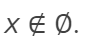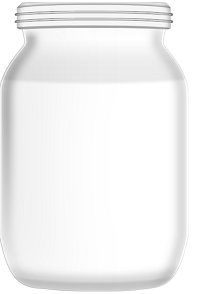# Empty Function, Empty Set: Definition, Examples

## What is an Empty Function?

The empty function is, literally, empty. There isn’t any need to state what elements are in the domain, because there are none! That said, if you wanted to write the empty function in notation, it’s written as

f: : ∅ → X

or sometimes

Empty = {}

Note that the symbol for an empty function or set (∅) is unique, has nothing to do with the number zero (0), and is unrelated to the Greek letter φ. It was first used by the Bourbaki group and actually came from the Norwegian alphabet (Craven, n.d.).

## Empty Function and the Empty Set

Empty functions are intertwined with empty sets (also called null sets). The empty function has a domain of the empty set, and is a graph of the empty set. Like the empty function, the empty set contains nothing.

It may seem bizarre that such a thing as an empty set exists. However, all of mathematics works off of the idea that this empty set does in fact exist as a concept.

Slightly more formally, we say that the empty set, ∅, is the set that for all x:The statement says that every “x” is not in the set ∅ which makes sense (sort of!) because if any x-values were in ∅ then the set wouldn’t be empty.

## The History of “Nothing”

The number zero “0” doesn’t actually exist (as an object) either. But it’s needed to make other math work. Although zero is very familiar to anyone studying any kind of math in modern times, it’s a relatively new invention (in the big scheme of things). Neither the Egyptians nor the Ancient Greeks have zero either as a name or as a placeholder.

The “zero” didn’t actually make it’s way to Europe until the 12th century, when the Italian mathematician Fibonacci wrote his “Abacus book” (Wallin, 2002). It’s a good thing that the idea gained traction, because without zeros, we wouldn’t have computers or any kind of electronics, which all work off the binary system of 1 (on) or 0 (off).

## Non Empty and Empty Sets

A nonempty set is a set that has one or more elements.

A “set” is just a well-defined collection of distinct objects, and their union—the set—is an object itself. A set can contain anywhere from 0 to an infinite number of items.

In math terminology, a set with 0 items is called the empty set. There is only one empty set. The nonempty set, then, is just as its name would suggest: a mathematical set that is not actually empty.

Sometimes the nonempty set is called the nonvoid set.

## Importance of the Nonempty Set

Although it may seem a very basic, uninteresting idea, nonvoid sets are actually highly important in the groundwork of mathematics, especially in fields like linear algebra, topology, set theory and vector analysis. In fact, the whole system of natural numbers (whole, non-negative numbers that we use to count) can be constructed from the concept of an empty set.Imagine you have an empty jar on a table. That’s an empty set. Place the jar into a box, and the jar is still empty. The box has one set: an empty set (i.e. the jar). As the box has a single set, the contents of the box has a cardinality of 1. Place the box into a larger box, and the cardinality of the larger box’s contents becomes 2.* You can continue placing more and more boxes around the original jar (a.k.a. the empty set), creating infinite cardinal numbers.

The box analogy doesn’t always work. For example, if { } = empty set, then { { } } has cardinality 1, but { { { } } } also has cardinality 1. On the other hand, the set { { }, {{ }} } has cardinality 2.

The first step in a proof is often to define a set as non empty. That’s because unintentionally allowing for an empty set might lead to nonsense results, in a way similar to how dividing by zero is impossible in basic arithmetic proofs.

## Examples of Nonempty Sets

The very smallest nonempty set is the set with just one element; this is called the singleton set. There are any number of singleton sets; One element may be anything: a number, an ordered pair, even an object. The set {1} is a nonempty singleton set. So is the set {(5,6)}, the set containing one vector {[1 4 5 7] }, and the set with just you in it {you}.

Since all sets except the one unique set containing 0 elements are nonempty, other non singleton examples include the real numbers, the integers, the positive integers, the set {5, 7, 8}, and the set of students at Missouri State University. The set B, containing two colored shapes, is a non-empty set.

A union of non-empty sets is also an empty set. An intersection or a subset of a non-empty set, though, may possibly be empty.

## Set Functions

A set function has a domain that is a collection of sets. In other words, the function’s input is a set; it usually outputs a number, but the output could technically be any mathematical object (e.g. another set, a matrix, or anything else used in mathematics).

## References

Craven, T. (n.d.). Earliest Uses of Symbols of Set Theory and Logic. Retrieved December 15, 2019 from: http://www.math.hawaii.edu/~tom/history/set.html
Dumas, B. & McCarthy, J. Transition to Higher Mathematics: Structure and Proof
Second Edition. Retrieved December 15, 2019 from: https://www.math.wustl.edu/~mccarthy/SandP2.pdf
Wallin, N. (2002). The History of Zero. Retrieved December 15, 2019 from: https://yaleglobal.yale.edu/history-zero

CITE THIS AS:
Stephanie Glen. "Empty Function, Empty Set: Definition, Examples" From StatisticsHowTo.com: Elementary Statistics for the rest of us! https://www.statisticshowto.com/empty-function/